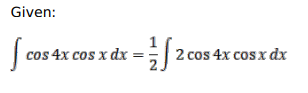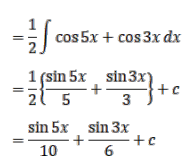# Solve this following

Question:

Mark $(\sqrt{)}$ against the correct answer in each of the following:

$\int \cos 4 x \cos x d x=?$

A. $\frac{1}{5} \sin 5 x+\frac{1}{3} \sin 3 x+C$

B. $\frac{1}{5} \cos 5 x-\frac{1}{3} \cos 3 x+C$

C. $\frac{1}{10} \sin 5 x+\frac{1}{6} \sin 3 x+C$

D. None of these

Solution: